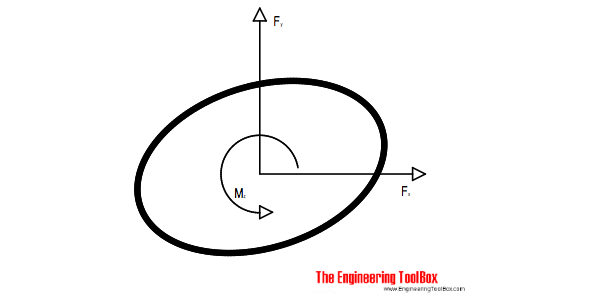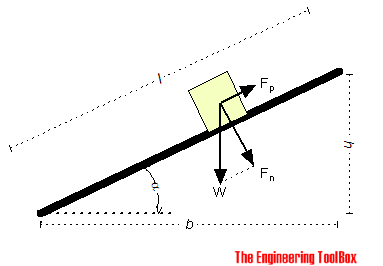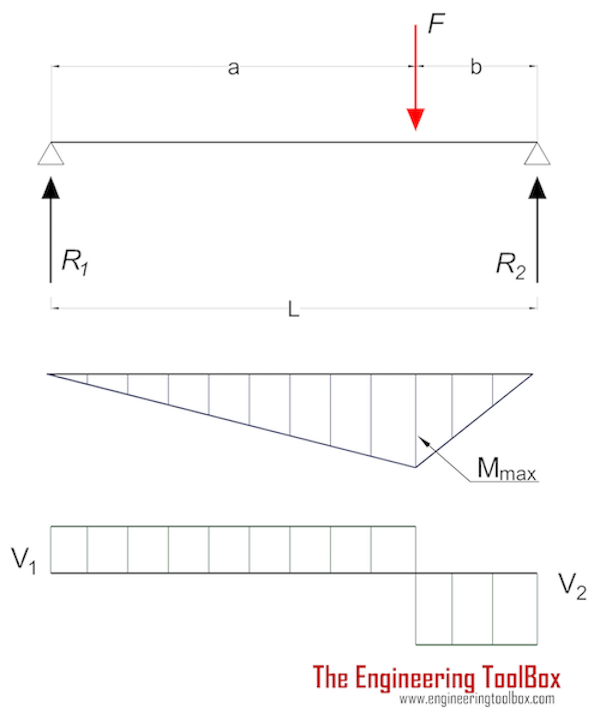Engineering ToolBox - Resources, Tools and Basic Information for Engineering and Design of Technical Applications!

# Support Reactions - Equilibrium

## Static equilibrium is achieved when the resultant force and resultant moment equals to zero.

Equilibrium of a body requires both a balance of forces to prevent the body from translating or having accelerated motion along a straight or curved path - and a balance of moments to prevent the body from rotating.Any static force system will be in equilibrium if the resultant force and resultant moment both are equal to zero.

Static equilibrium in a three dimensional system can be expressed as

ΣF = ΣFx = ΣFy = ΣFz = 0                          (1)

ΣM = ΣMx = ΣMy = ΣMz = 0                          (2)

where

F = force (N, lb)

M = moment (Nm, ft lb)

x, y, z = orthogonal axes

Often the loading of a body can be simplified to a two dimensional system with co-planar forces in the x-y plane.  Eq. 1 and 2 can  be reduced to

ΣF = ΣFx = ΣFy = 0                          (3)

ΣM = ΣMz = 0                          (4)

The best way to account for all forces acting on a body is to draw the body's free-body diagram. A free-body diagram shows the relative magnitude and direction of all forces acting upon an object in a given situation.Free-body diagram example - gravity and friction forces acting on a body on an inclined plane

### Example - Support Reactions on a Beam with Eccentric LoadA beam with length 6 m has an eccentric load of 9000 N 4 m from support 1. Applying the equations of equilibrium we have

Fx = R1x = R2x = 0                           (5)

Fy - (R1y + R2y) = 0                           (6)

M1 = Fy a - R2y (a + b) = 0                   (7)

Rearranging (7) to express R2y

R2y = F a / (a + b)                         (7b)

Eq. (7b) with values

R2 = (9000 N) (4 m) / ((4 m) + (2 m))

= 6000 N

= 6 kN

Rearranging (6) for R1y

R1y = Fy - R2y                        (6b)

Eq. (6b) with values

R1y = (9000 N) - (6000 N)

= 3000 N

= 3 kN

### Example - Reaction Forces on a Structural FrameA weight F (1000 N) is hanging in a structural frame as shown in the figure above. A structural analyses can be done with the following equations:

∑MA = RBy (230 cm)  - (1000 N) (100 cm) = 0                (1)

∑MB = RAy (230 cm) - (1000 N) (130 cm) = 0                (2)

∑MC-B = RBy (130 cm) - RBx h = 0                (3)

F = RAy + RBy                            (4)

RAx = RBx                           (5)

Eq. 1 can be rearranged to

RBy = (1000 N) (100 cm) / (230 cm)

= 435 N

Eq. 2 can be rearranged to

RAy = (1000 N) (130 cm) / (230 cm)

= 565 N

The height h in eq. 3 can be calculated as

h = (1532 - 1302)1/2

= 80.7 cm

Eq. 3 can then be rearranged to

RBx = (435 N) (130 cm) / (80.7 cm)

= 700 N

From eq. 5

RAx  = RBx

= 700 N

## Related Topics

• ### Mechanics

Forces, acceleration, displacement, vectors, motion, momentum, energy of objects and more.
• ### Statics

Loads - forces and torque, beams and columns.

## Related Documents

• ### Acceleration of Gravity and Newton's Second Law

Acceleration of gravity and Newton's Second Law - SI and Imperial units.
• ### Bodies Moving on Inclined Planes - Acting Forces

Required forces to move bodies up inclined planes.
• ### Force

Newton's third law - force vs. mass and acceleration.
• ### Impulse and Impulse Force

Forces acting a very short time are called impulse forces.
• ### Mass Moment of Inertia

The Mass Moment of Inertia vs. mass of object, it's shape and relative point of rotation - the Radius of Gyration.
• ### Mass vs. Weight

Mass vs. weight - the Gravity Force.
• ### Torque or Moment of Force - Online Converter

Torque or moment - the tendency of a force to rotate an object.

## Engineering ToolBox - SketchUp Extension - Online 3D modeling!

Add standard and customized parametric components - like flange beams, lumbers, piping, stairs and more - to your Sketchup model with the Engineering ToolBox - SketchUp Extension - enabled for use with older versions of the amazing SketchUp Make and the newer "up to date" SketchUp Pro . Add the Engineering ToolBox extension to your SketchUp Make/Pro from the Extension Warehouse !

We don't collect information from our users. More about

## Citation

• The Engineering ToolBox (2005). Support Reactions - Equilibrium. [online] Available at: https://www.engineeringtoolbox.com/equilibrium-d_943.html [Accessed Day Month Year].

Modify the access date according your visit.

9.27.11